Click to Chat

1800-1023-196

+91-120-4616500

CART 0

• 0

MY CART (5)

Use Coupon: CART20 and get 20% off on all online Study Material

ITEM
DETAILS
MRP
DISCOUNT
FINAL PRICE
Total Price: Rs.

There are no items in this cart.
Continue Shopping```Objective Type Questions

42. Total number of parallel tangents of f1(x) = x2 – x + 1 and f2(x) = x3 – x2 –2x + 1 is equal to

(A) 2                                           (B) 3

(C) 4                                           (D) None of these

Solution:

(D) f1’ (x1) = 2x1 – 1, f2’(x2) = 3x22 – 2x2 – 2 .

Let tangents drawn to the curves y = f1(x) and y = f2(x) at (x1, f(x1)) and (x2, f(x2)) are parallel, then 2x1 – 1 = 3x22 – 2x2 – 2, which is possible for infinite number of order pair (x1, x2).

43. The function 2tan3x – 3tan2x + 12tanx + 3, x Î  is

(A) Increasing

(B) Decreasing

(C) Increasing in (0, p/4) and decreasing in (p/4, p/2)

(D) None of these

Solution:

(A)       Let f(x) = 2 tan3x – 3tan2x + 12 tanx + 3

f'(x) = (6tan2x – 6tanx + 12) sec2x

= 6sec2x (tan2x – tanx + 2) > 0

Hence f(x) is always increasing.

44. Let f (x) = (4–x2)2/3 , then f has a

(A) a local maxima at x = 0                   (B) a local maxima at x = 2

(C) a local maxima at x = –2                 (D) none of these

Solution:

(A)       f(x) = (4 – x2)2/3

f'(x) = –4x / 3(4–x2)1/3 = 4x / 3(x2–4)1/3

at x = –2         local minima

x = 0            local maxima

x = 2            local minima

45. Let f (x) = x3 – 6x2 + 9x + 18, then f (x) is strictly decreasing in

(A) (–∞, 1]                                             (B) [3, ∞)

(C) (–∞, 1] U [3, ∞)                                 (D) [1, 3]

Solution:

(D)       f(x) = x3 – 6x2 + 9x + 18

f'(x) = 3x2 – 12x + 9

= 3(x2 – 4x + 3)

= 3(x – 1)(x – 3) £ 0

x ∈ [1, 3]

46. The absolute minimum value of x4 – x2 – 2x+ 5

(A) is equal to 5                                    (B) is equal to 3

(C) is equal to 7                                    (D) does not exist

Solution:

(B)       f(x) = x4 – x2 – 2x + 5

f'(x) = 4x3 – 2x – 2

= (x – 1) (4x2 + 4x + 2)

Clearly at x = 1, we will set the minimum value which is 3.

47. Equation of the tangent to the curve y = e–|x| at the point where it cuts the line x=1

(A) is ey + x =2                   (B) is x + y = e

(C) is ex + y = 1                  (D) does not exist

Solution:

(A) y = e–|x| cut the line x = 1 at (1, 1/e)

(dy/dx)(11,1/e) = –1/e

Tangent y = –1/e  = –1/e (x – 1)

=>        ey + x = 2

48. Rolle’s theorem holds for the function x3 + bx2 + cx, 1 < x < 2 at the point 4/3, the value of b and c are;

(A) b = 8, c = - 5                       (B) b = -5, c = 8

(C) b = 5, c = -8                        (D) b = -5, c = -8.

Solution:

(D)       f'(4/3) = 0

=>        16 + 8b + 3c = 0

Also f(1) = f(2)

=>        3b + c + 7 = 0

Hence b = – 5, c = –8

49. The number of value of k for which the equation x3 – 3x + k = 0 has two different roots lying in the interval (0, 1) are

(A) 3                                          (B) 2

(C) infinitely many                         (D) no value of k satisfies the requirement.

Solution:

(C)       Let f(x) = x3 – 3x + k

f'(x) = 3(x – 1) (x + 1)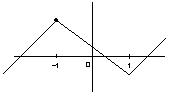only are root may lie between –1 and 1

50. From mean value theorem: f(b) – f(a) = (b –a) f' (x1); a < x1 < b if f(x) = 1/x , then x1 =

(A) √ab                          (B) a+b/2

(C) 2ab/q+b                    (D) b–a/b+a

Solution:

(A)       Since f(x) = 1/x

f'(x1) = f(b) – f(a) / b – a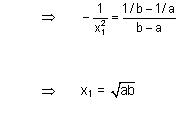13.1    OBJECTIVE LEVEL – 2

51. The minimum value of ax + by, where xy = r2, is (r, ab >0)

(A) 2r √ab                                (B) 2ab√r

(C)       –2r √ab                        (D) None of these

Solution:

(A)       Let f(x) = ax + br2 / x

f ’(x) = a – br2 / x = 0

x = √b / √a r

f (√b / √a r) = a√br / √a + √br2 / √br √a = 2r √ab

52. If a, b, c, d are four positive real numbers such that abcd =1, then minimum value of (1+ a) (1 + b) (1 + c) (1 + d) is

(A) 8                                    (B) 12

(C) 16                                   (D) 20

Solution:

(C)       Applying AM ³GM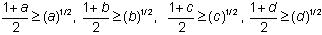Multiplying all focus (1 + a) (1 + b)(1 + c)(1 + d) ³ 16

53. A lamp of negligible height is placed on the ground ‘l1’ metre away from a wall. A man ‘l2’ metre tall is walking at a speed of l1/10 m/sec. from the lamp to the nearest point on the wall. When he is mid-way between the lamp and the wall, the rate of change in the length of this shadow on the wall is

(A) –5l2 / 2 m/sec.                   (B) –2l2 / 5 m/sec.

(C) –l2 / 2 m/sec.                     (D) –l2 / 5 m/sec.

Solution:

(B)       Clearly h / l1 = l2 / l1 – x

=> hl1 – hx = l1l2        (Since h is decreasing put a – ve sign)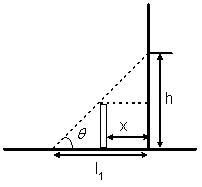at mid point x1 = l1/2, h = 2l2

=> dh/dt = 2l2/5

f ’(x) = 2ex + ae-x + 2a + 1> 0 clear a > 0

54. Let f (x) =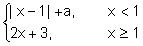. If f (x) has a local minima at x = 1, then

(A) a > 5                          (B) a > 5

(C)       a > 0                    (D) none of these

Solution:

(A) f (x) =Local minimum value of f (x) at x = 1, will be 5

i.e. 1 –x + a ³ 5 at x = 1 => a ³ 5

55. Global minimum value of f (x) = x8 + x6 –x4 –2x3 –x2 –2x + 9 is

(A) 0                                 (B) 1

(C) 5                                 (D) 9

Solution:

(C) We will get the minimum value of f(x) at x = 1 which is 5

```### Course Features

• 731 Video Lectures
• Revision Notes
• Previous Year Papers
• Mind Map
• Study Planner
• NCERT Solutions
• Discussion Forum
• Test paper with Video Solution